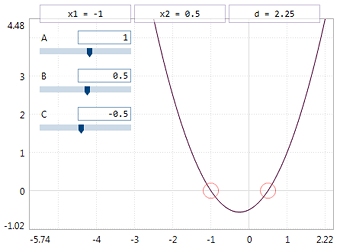## parabolaPlot and roots of the quadratic equation: A*x2 + B*x + C = 0

The project makes a plot of y = A*x2 + B*x + C function and calculates its discriminant and roots. If A = 0 then the linear equation is solved and corresponding linear function plotted. If A = 0 and B = 0 then the constant function y = C is plotted with no attempts of finding the roots, of course.
The values of A, B and C coefficients are set with the sliders (you can change ranges inside the code). Use these sliders to see how each of the coefficients affects the function plot.
Note, how the root values and the plot updates are implemented: the `update` function is launched on each change of the slider values and on each change of displayed range (e.g. when the zoom is changed or the graphics window is scrolled).

Remember to switch on the coordinate system in the POOL options (Display as a plot).